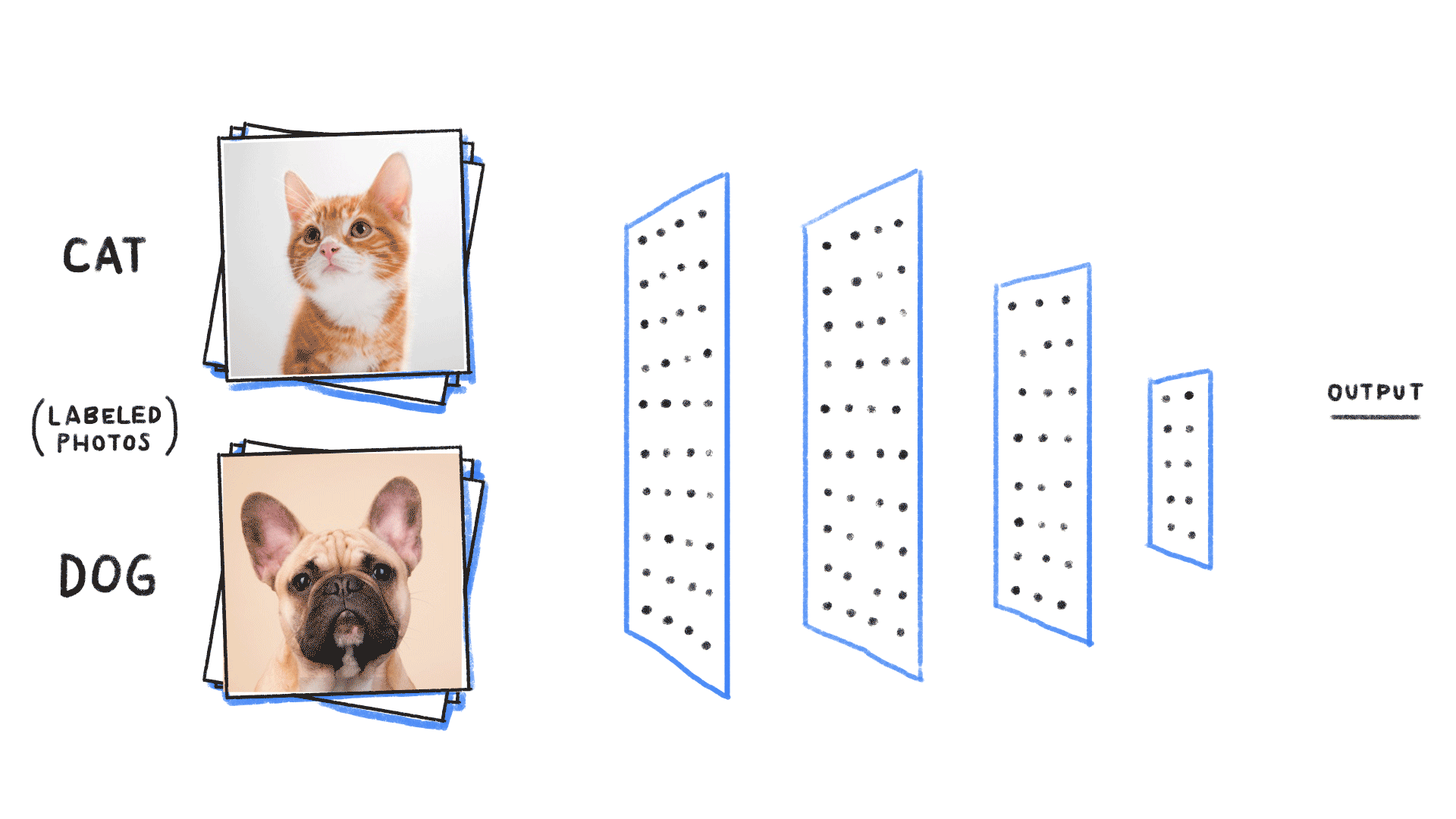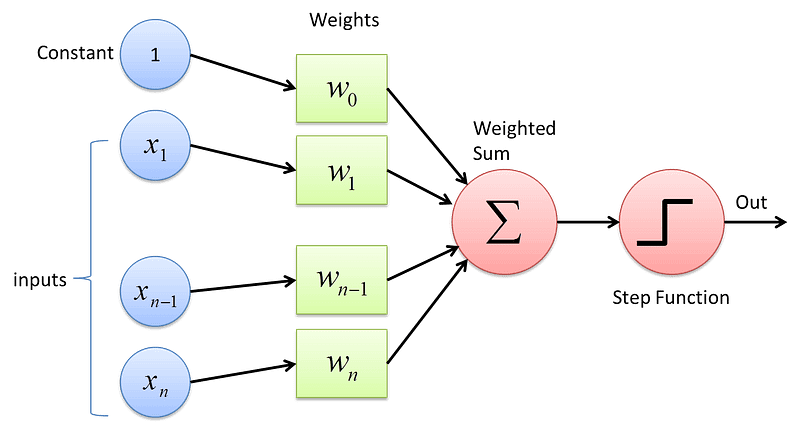# 自然语言

## 起源时期

• 信息的冗余提高信息的安全性。
• 语言的数据（语料）对翻译至关重要。

• 中国：个十百千万亿兆（大端
• 罗马：$IVXM$（大端相减，小端相加）
• 印度：0123456789（隐式进制单位）

## 转折时期

• 计算机能否处理自然语言？Yes。
• 处理方法是否与人一样？Yes。

1950-170。用电脑模拟人脑。成果几乎为0。

1970-21世纪。基于数学模型+统计方法。实质性突破。

• 应用层：语音识别，机器翻译，自动问答
• 认知层：自然语言处理
• 基础层：句法分析，语义分析

• 算法复杂度——上下文无关算法：$O(n^2)$；上下文相关算法：$O(n^6)$。

## 统计模型

### 通用模型

• 美联储主席本·伯南克昨天告诉媒体7 000 亿美元的救助资金将借给上百家银行、保险公司和汽车公司。

• 本·伯南克美联储主席昨天7 000 亿美元的救助资金告诉媒体将借给银行、保险公司和汽车公司上百家。

• 联主美储席本·伯诉体南将借天的救克告媒昨助资金70 元亿00 美给上百百百家银保行、汽车险公司公司和。

（注：为避免值太小，可以使用对数概率）

• 假设任意一个词出现的概率只同它前面的词有关。（前驱，联想链表）（n元模型的复杂度为$O(|V|^n)$，$V$是词汇量）

$r$较大时，

• 统计中频率$\approx$概率。

$r$较小时，我们利用Good-Turing估计，将$r$较小的词的概率进行修正

• 一般$N_r$和$r$的关系呈现凹性负相关Zipf定律
因而$r$ 越小，$N_r$上升越快，故我们修正得到的$d_r$会比$r$小得多。（并且，有正定性：$d_0>0$）
这样，我们实际上只把一些中等频率的词的概率分配给了生僻词。（卡茨退避法

### 分词

#### 动态规划+统计

dp[i,j] j0 1 2 3 4
i0 0.05 0.1 0.4
1 0.01
2 0.02 0.2
3 0.04 0.15
4 0.03

dp[i,j] j0 1 2 3 4
i0 0.05 0.1 0.4 0.44 0.55
1 0.01 0.03 0.21 0.24
2 0.02 0.2 0.23
3 0.04 0.15
4 0.03

#### Viterbi算法（相比穷举的指数级复杂度：$O((D^2)^N)=O(D^N)$优化不少）

（我的思考）

• BFS只能无偏地传播，搜索进度慢，形成比较均衡的搜索树
• DFS只能定向传播（又称为传递，因为仿佛有一个虚拟的信物/令牌在节点之间传递），容易陷入过于细分的领域，运气成分高，并且容易形成强烈失衡的搜索树
• FFS综合了BFS和DFS，充分利用已知信息（通常信息集中于前几层），引入概率模型，在每一次搜索时选择概率最大的多个进行搜索，实现适度均衡的搜索树。（与剪枝策略相反）
（在有限传播中可能找不到类似梯度这样局部的全面、精准的度量。有限传播算法考虑在有限资源的前提最大可能程度地获得答案）
• 类似算法：A*算法。（More，启发式搜索算法）

• 频分多址（FDMA）：将不同的频率（频道）一一对应地分给用户。
• 时分多址（TDMA）：类似时间片轮转，将同一频道切分为细小的时间片给不同用户。当切分得足够小，可近似具有实时性。
• 码分多址（CDMA）：类似量子态叠加（或者Fourier解析），用户通过一个密匙从大量的信息中过滤出自己的信号。

### 隐马尔可夫• 预测）给定模型，计算特定O序列的概率【Forward-Backward算法
• 溯源）给定模型+特定O序列，估计最佳似然S序列【Viterbi算法
• 训练给定观测数据，训练隐马尔可夫模型参数Baum-Welch算法

Baum-Welch算法将试图找到其中最可能的模型$M_{\theta}$（最佳模型）。

Baum-Welch算法（迭代）：

• 首先找到任意一个满足O序列的HMM模型$M_i$
• 通过$M_1$可以利用O序列反向生成一个标注数据集
• 利用标注数据集，根据隐马尔可夫（解码算法）生成新的模型$M_{i+1}​$
• 再次反向生成、生成……
• ……
• 直到收敛。找到质量足够好的模型$M_n$（期望最大化
• 用$Mn近似表示最佳模型M{\theta}$。

# 信息学

## 信息论

• 信息熵：$H(X)=-\sum_{x\in X}P(x)logP(x)$。

• 条件熵：$H(X|Y)=-\sum_{x\in X,y\in Y}P(x,y)logP(x|y)$。

$H(X)\geq H(X|Y)$。（可以推广。意味着n元模型的精确度高于n-1元模型）

• 互信息：$I(X;Y)=\sum_{x\in X,y\in Y}P(x,y)log \cfrac{P(x,y)}{P(x)P(y)}$。

$I(X;Y)=H(X)-H(X|Y)$。

• 交叉熵（相对熵）：$KL(f(x)\mid \mid g(x))=\sum_{x\in X}f(x)\cdot log\cfrac{f(x)}{g(x)}$。

 Thomas M. Cover与Joy A. Thomas著的无比经典的教材《Elements of Information Theory》（中文版书名为《信息论基础》，阮吉寿、张华等译）；
 Abbas El Gamal和Young-Han Kim合著的《Network Information Theory》（中文版书名为《网络信息论》，张林译）；
 Imre Csiszár和János Körner Csizar所著的学院派经典《Information Theory: Coding Theorems for Discrete Memoryless Systems》。

## 信息指纹

### 集合判等

• 暴力法：两两做比较，$O(N^2)$
• 排序法：先排序，再从头至尾比较，$O(NlogN)$
• 散列法：将第一个集合放到散列表中，然后拿第二个集合作对比，$O(N)$。但需要额外的$O(N)$空间。
• 指纹法：对集合$S$，定义指纹$FP(S)=FP(e_1)+FP(e_2)+…+FP(e_n)$。比较即可。就地算法。

#### 反盗版（视频）

• 关键帧提取
• 尽管视频每秒可能有几十帧，但每一帧的差异不大。一般来说，每数秒才能提取出一个关键帧。
• 特征提取
• 利用信息指纹来表示关键帧，退化为集合判等问题

（我的解法）

### 相似哈希

• 扩展【将8位指纹处理为8个实数$r_i$】

• 经过上面的处理，我们将获得一个实数向量$R={r_i}$。

• 收缩【将8个实数$r_i$重新布尔化为8位指纹】

• 我们获得了可以进行相似性比较的信息指纹$P$（相似Hash）。
若两个网站雷同，则相似哈希接近。

## 布隆过滤器

• 对每一个邮件地址$X$，用8个随机数产生器$F_i$先生成8个信息指纹$f_i$
• 然后将$f_i$用随机数产生器$G$将$f_i$映射到1~16亿的8个自然数$g_i$
• 将$g_i$对应的bit位设置为1
• 对1亿个电子邮件地址做相同处理

• 用$F_i$转换该地址，生成信息指纹$s_i$
• 将$s_i$用$G$映射到8个bit位，若对应为1，则命中

## 密码学

• 无论获取多少密文，也无法消除己方情报系统的不确定性。
为了这个目的，不仅要密文之间相互无关，同时密文还是看似完全随机的序列

# 搜索

## 网络爬虫

### 网络爬虫的构建

1. BFS或DFS
1. 小容量爬虫，爬取首页即可，用BFS
2. 考虑握手（和网站建立连接）成本，在特定的网站适当采用DFS
2. 页面分析和URL（链接）提取
1. 通常采用队列，直接提取HTML中的URL标签
2. 若网站采用了不规范的脚本（如JaveScript），需要通过高级模拟手段，利用浏览器内核解析网页
3. URL记录表
1. 用一个散列表记录已经遍历过的网页（分摊复杂度$O(1)$）
2. 分布式系统散列表的维护和访问是难题

## PageRank

### 质量

• 收录网页少，只能对常见词索引
• 查询的结果相关性差

PageRank是一种整体化思维

### 相关性

• 用户的点击数据
• 完备的索引
• 网页质量的度量（PageRank）
• 用户偏好
• 网页与查询的相关性

#### TF-IDF

TF：Term Frequency，词频。

IDF：Inverse Document Frequency，逆文本频率指数。

### 权威性

• 对网页标题、正文进行句法分析，获取Mention信息
• 利用互信息，找到主题短语和信息源的相关性
• 对主题短语进行聚类（可用矩阵奇异值分解）
• 对网页进行聚类，权威性的度量只能建立在子域（Subdomain）或子目录（Subdirectory）等粗略的级上。

#### 新闻分类

• 充分利用TF-IDF向量中的非零元素
• 删除虚词/停止词（消除了噪声）
• 考虑词的位置加权（比如，在标题、首尾进行额外加权）

#### 奇异值分解

$X_{M\times r}$：每一行（M）代表一个词，每一列（r）表示一个语义相近的词类。（元素值代表相关度）

$Y_{r\times N}$：每一列（N）代表一篇文本， 每一行（r）对应一个主题。

$B_{r\times r}$：表示词类和文章类（主题）的相关性。

## 地图定位

• 利用卫星定位
• 地址的识别
• 根据起点和终点，规划最短或最快路线

## SEO与作弊

SEO就是搜索引擎优化。

SEO一般要适当，遵循搜索引擎的规则。

• 重复关键词
• 利用TF的相对词频
• 买卖链接
• 识别链接的流通

• 从信息源出发，加强通信（编码）自身的抗干扰能力
• 利用相反的信号抵消噪音
• 搜集一段时间的作弊信息后，还原其原有的排名
• 从传输来看，过滤掉噪音，还原信息

• 互相链接的节点称为Clique，发现Clique并直接应用到反作弊中

• 对于使用JavaScrpt跳转页面，其落地页（Landing Page）质量非常高，但进入之后会立即通过JS程序被跳转到商业网站

## 搜索广告

• 竞价排名（Overture、百度）
• 利用经验预估点击率（Click Through Rate，CTR），预测用户的点击概率
• 逻辑回归模型${\beta_i}$向量就是该模型的参数向量。可以通过神经网络训练得到。比如最大熵模型的$GIS$或者$IIS$算法。

# 数学模型

• 一个正确的模型应当在形式上是简单的
• 一个正确的模型最开始可能并不如复杂的错误模型准确
• 大量的准确数据对研发很重要
• 正确的模型可能受到噪声干扰，此时应坚定信心，积极找寻噪声源

## 最大熵模型

$P(w_3|w_1,w_2,s)=\cfrac{1}{Z(w_1,w_2,s)}e^{\lambda_1(w_1,w_2,w_3)+\lambda_2(s,w_3)}$.
（$Z$是归一化因子，保证$P$概率归一，与参数$\lambda_i$一起需要被训练出来）

（参数$\lambda_i$通过模型的训练得到）

• 假定第零次迭代的初始模型为等概率的均匀分布（类似PageRank的设定）
• 用第N次迭代的模型来估算每种信息特征在训练数据中的分布，比较并调节相应模型参数。
• 重复，直至收敛。

GIS算法是一个典型的期望值最大化算法（Expectation Maximization，EM）。

GIS收敛缓慢，而且不稳定，因此很少有人真的使用。

## 条件随机场

• 一是指根据文法对一个句子进行分析，建立其语法树，即文法分析（Synatactic Parsing）；
• 二是指对一个句子中各成分的语义进行分析，得到对这个句子语义的一种描述（语义树），即语义分析（Semantic Parsing）。

Eugene Charniack提出原则：选择文法规则，让被分析的句子的语法树概率达到最大。

• 对任意一个句子进行分词
• 美联储|主席|本·伯南克|昨天|告诉|媒体|7 000 亿|美元|的|救助|资金|将|借给|上百|家|银行|、|保险公司|和|汽车公司
• 扫描（从左往右），整合出高一阶的词组
• （美联储主席）|本·伯南克|昨天|告诉|媒体|（7 000 亿美元）|的|（救助资金）|（将借给）|（上百家）|（银行、保险公司和汽车公司）
• 重复扫描，整合……
• 【美联储主席本·伯南克】|昨天|告诉|媒体|【7 000 亿美元的救助资金】|（将借给）|【上百家银行、保险公司和汽车公司】
• 直到仅剩一个括号为止

## 贝叶斯网络

### 人工神经网络• 所有节点分层，每一层通过有向弧指向上一层节点，但同一层无弧连接，不跨层连接
• 每条弧有一个权重
• 完了

#### 感知机Minsky指出)：感知机无法解决异或问题。（More

#### 多层感知机## 期望最大化

• 利用事先设定好的类别对新的文本进行分类
• 需要事先设定好类别和文本中心（Centroids）
• 自底向上地将文本两两比较进行聚类
• 计算时间较长

• 随机挑选类别的中心，然后反复优化直到收敛
• 要利用到文本TF-IDF向量和余弦算法

Baum-Welch算法（隐马尔可夫链），GIS算法（最大熵模型）都是典型的EM算法。

EM算法的分布描述：

• E过程（Expectation）：根据已有模型，计算观测数据输入到模型的结果
• Baum-Welch：计算每个状态转移的次数和输出的次数
• GIS：计算每一个特征的数学期望值
• M过程（Maximization）：重新计算模型参数，以最大化期望
• Baum-Welch：根据这些次数重新估计隐含马尔可夫模型的参数
• GIS：根据数学期望值和实际观测值的比，调整最大熵模型参数

EM算法优化的目标函数必须是一个凸函数，才能确保得到全局最优解。

0%